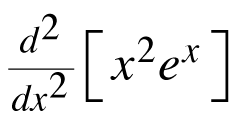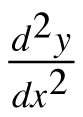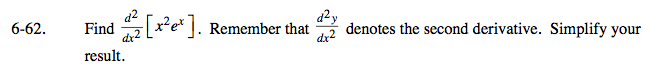### Home > CALC > Chapter 6 > Lesson 6.2.1 > Problem6-62

6-62.

Find. Remember thatdenotes the second derivative. Simplify your result. Homework Help ✎Use the Product Rule to find the derivative, and then derive again to find the second derivative.

$\frac{d}{dx}=(x^2)^\prime e^x+x^2(e^x)^\prime=2xe^x+x^2e^x=e^x(2x+x^2)$